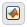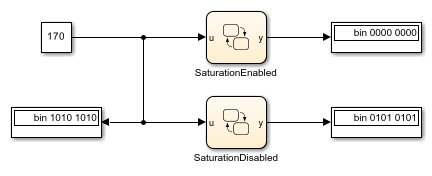## Supported Operations for Chart Data

Stateflow® charts in Simulink® models have an action language property that defines the operations that you can use in state and transition actions. The language properties are:

•MATLAB® as the action language.

•C as the action language.

For more information, see Differences Between MATLAB and C as Action Language Syntax.

### Binary Operations

This table summarizes the interpretation of all binary operations in Stateflow charts according to their order of precedence (0 = highest, 10 = lowest). Binary operations are left associative so that, in any expression, operators with the same precedence are evaluated from left to right. The order of evaluation for other operations is unspecified. For example, in this assignment

`A = f() > g();`
the order of evaluation of `f()` and `g()` is unspecified. For more predictable results, it is good coding practice to split expressions that depend on the order of evaluation into multiple statements.

Operation

Precedence

MATLAB as the Action Language

C as the Action Language

`a ^ b`

0

Power.

Power. This operation is equivalent to the C library function `pow`. Operands are first cast to floating-point numbers. For more information, see Call C Library Functions.

Enable this operation by clearing the Enable C-bit operations chart property. For more information, see Enable C-bit operations.

`a * b`

1

Multiplication.

Multiplication.

`a / b`

1

Division.

Division.

`a %% b`

1

Not supported. Use the `rem` or `mod` function.

Remainder. Noninteger operands are first cast to integers.

`a + b`

2

`a - b`

2

Subtraction.

Subtraction.

`a >> b`

3

Not supported. Use the `bitshift` function.

Shift `a` to the right by `b` bits. For more information, see Bitwise Operations.

`a << b`

3

Not supported. Use the `bitshift` function.

Shift `a` to the left by `b` bits. For more information, see Bitwise Operations.

`a > b`

4

Comparison, greater than.

Comparison, greater than.

`a < b`

4

Comparison, less than.

Comparison, less than.

`a >= b`

4

Comparison, greater than or equal to.

Comparison, greater than or equal to.

`a <= b`

4

Comparison, less than or equal to.

Comparison, less than or equal to.

`a == b`

5

Comparison, equal to.

Comparison, equal to.

`a ~= b`

5

Comparison, not equal to.

Comparison, not equal to.

`a != b`

5

Not supported. Use the operation ```a ~= b```.

Comparison, not equal to.

`a <> b`

5

Not supported. Use the operation ```a ~= b```.

Comparison, not equal to.

`a & b`

6

Logical AND. For bitwise AND, use the `bitand` function.

• Bitwise AND (default). Enable this operation by selecting the Enable C-bit operations chart property.

• Logical AND. Enable this operation by clearing the Enable C-bit operations chart property.

`a ^ b`

7

Not supported. For bitwise XOR, use the `bitxor` function.

Bitwise XOR (default). Enable this operation by selecting the Enable C-bit operations chart property. For more information, see Bitwise Operations and Enable C-bit operations.

`a | b`

8

Logical OR. For bitwise OR, use the `bitor` function.

• Bitwise OR (default). Enable this operation by selecting the Enable C-bit operations chart property.

• Logical OR. Enable this operation by clearing the Enable C-bit operations chart property.

`a && b`

9

Logical AND.

Logical AND.

`a || b`

10

Logical OR.

Logical OR.

### Unary Operations and Actions

This table summarizes the interpretation of all unary operations and actions in Stateflow charts. Unary operations:

• Have higher precedence than the binary operators.

• Are right associative so that, in any expression, they are evaluated from right to left.

Operation

MATLAB as the Action Language

C as the Action Language

`~a`

Logical NOT. For bitwise NOT, use the `bitcmp` function.

• Bitwise NOT (default). Enable this operation by selecting the Enable C-bit operations chart property.

• Logical NOT. Enable this operation by clearing the Enable C-bit operations chart property.

`!a`

Not supported. Use the operation `~a`.

Logical NOT.

`-a`

Negative.

Negative.

`a++`

Not supported. Use the expression ```a = a+1```.

Increment. Equivalent to `a = a+1`.

`a--`

Not supported. Use the expression ```a = a-1```.

Decrement. Equivalent to `a = a-1`.

### Assignment Operations

This table summarizes the interpretation of assignment operations in Stateflow charts.

Operation

MATLAB as the Action Language

C as the Action Language

`a = b`

Simple assignment.

Simple assignment.

`a := b`

Not supported. Use type cast operations to override fixed-point promotion rules. See Type Cast Operations.

Assignment of fixed-point numbers. See Override Fixed-Point Promotion in C Charts.

`a += b`

Not supported. Use the expression ```a = a+b```.

Equivalent to `a = a+b`.

`a -= b`

Not supported. Use the expression ```a = a-b```.

Equivalent to `a = a-b`.

`a *= b`

Not supported. Use the expression ```a = a*b```.

Equivalent to `a = a*b`.

`a /= b`

Not supported. Use the expression ```a = a/b```.

Equivalent to `a = a/b`.

`a &= b`

Not supported. Use the expression ```a = bitand(a,b)```.

Equivalent to `a = a&b` (bitwise AND). Enable this operation by selecting the Enable C-bit operations chart property. For more information, see Bitwise Operations and Enable C-bit operations.

`a ^= b`

Not supported. Use the expression ```a = bitxor(a,b)```.

Equivalent to `a = a^b` (bitwise XOR). Enable this operation by selecting the Enable C-bit operations chart property. For more information, see Bitwise Operations and Enable C-bit operations.

`a |= b`

Not supported. Use the expression ```a = bitor(a,b)```.

Equivalent to `a = a|b` (bitwise OR). Enable this operation by selecting the Enable C-bit operations chart property. For more information, see Bitwise Operations and Enable C-bit operations.

### Type Cast Operations

To convert a value of one type to a value of another type, use type cast operations. You can cast data to an explicit type or to the type of another variable.

#### Cast to Explicit Data Type

To cast a numeric expression to an explicit data type, use a MATLAB type conversion function of the form:

```<type_fun>(expression) ```

`<type_fun>` is a type conversion function that can be `double`, `single`, `int32`, `int16`, `int8`, `uint32`, `uint16`, `uint8`, or `fi` (Fixed-Point Designer). `<type_fun>` can also be `boolean`, `int64`, or `uint64`. For example, this statement casts the expression `x+3` to a 16-bit unsigned integer and assigns its value to the data `y`:

```y = uint16(x+3) ```

Alternatively, in charts that use MATLAB as the action language, you can use the `cast` function with a type keyword `<type_key>`:

```cast(expression,<type_key>) ```

Type keywords include `"double"`, `"single"`, `"int64"`, `"int32"`, `"int16"`, `"int8"`, `"uint64"`, `"uint32"`, `"uint16"`, and `"uint8"`. For instance, as in the preceding example, this statement casts the expression `x+3` to a 16-bit unsigned integer and assigns it to `y`:

```y = cast(x+3,"uint16") ```

#### Cast Type Based on Other Data

To make type casting easier, you can convert the type of a numeric expression based on the types of other data.

In charts that use MATLAB as the action language, call the `cast` function with the keyword `"like"`. For example, this statement converts the value of `x+3` to the same type as that of data `z` and assigns it to `y`:

```y = cast(x+3,"like",z) ```
In this case, the data `z` can have any acceptable Stateflow type.

In charts that use C as the action language, the `type` operator returns the type of an existing Stateflow data. Use this return value in place of an explicit type in a `cast` operation. For example, this statement converts the value of `x+3` to the same type as that of data `z` and assigns it to `y`:

```cast(x+3,type(z)) ```

### Bitwise Operations

This table summarizes the interpretation of all bitwise operations in Stateflow charts that use C as the action language.

Operation

Description
`a & b`Bitwise AND.
`a | b`Bitwise OR.
`a ^ b`Bitwise XOR.
`~a`Bitwise NOT.

`a >> b`

Shift `a` to the right by `b` bits.

`a << b`

Shift `a` to the left by `b` bits.

Except for the bit shift operations `a >> b` and ```a << b```, you must enable all bitwise operations by selecting the Enable C-bit operations chart property. See Enable C-bit operations.

Bitwise operations work on integers at the binary level. Noninteger operands are first cast to integers. Integer operands follow C promotion rules to determine the intermediate value of the result. This intermediate value is then cast to the type that you specify for the result of the operation.

Note

Bitwise operations are not supported in charts that use MATLAB as the action language. Instead, use the functions `bitand`, `bitor`, `bitxor`, `bitnot`, or `bitshift`.

#### Bitwise Operations and Integer Overflows

The implicit cast used to assign the intermediate value of a bitwise operation can result in an overflow. To preserve the rightmost bits of the result and avoid unexpected behavior, disable the chart property Saturate on Integer Overflows.

For example, both charts in this model compute the bitwise operation `y = ~u`. The charts compute the intermediate value for this operation by using the target integer size of 32 bits, so the 24 leftmost bits in this value are all ones. When the charts assign the intermediate value to `y`, the cast to `uint8` causes an integer overflow. The output from each chart depends on how the chart handles integer overflows.

• If Saturate on Integer Overflow is enabled, the chart saturates the result of the bitwise operation and outputs a value of zero.

• If Saturate on Integer Overflow is disabled, the chart wraps the result of the bitwise operation and outputs its eight rightmost bits.This table summarizes the interpretation of pointer and address operations in Stateflow charts that use C as the action language.

Operation

Description

`&a`

Address operation. Use with custom code and Stateflow variables.

`*a`

Pointer operation. Use only with custom code variables.

For example, the model `sf_bus_demo` contains a custom C function that takes pointers as arguments. When the chart calls the custom code function, it uses the `&` operation to pass the Stateflow data by address. For more information, see Integrate Custom Structures in Stateflow Charts.

Pointer and address operations are not supported in charts that use MATLAB as the action language. Pointers to structures should only be used in read-only mode and are only valid during the call in which they are passed.

### Replace Operations with Application Implementations

If you have Embedded Coder® or Simulink Coder™, you can configure the code generator to apply a code replacement library (CRL) during code generation. The code generator changes the code that it generates for operations to meet application requirements. With Embedded Coder, you can develop and apply custom code replacement libraries.

Operation entries of the code replacement library can specify integral or fixed-point operand and result patterns. You can use operation entries for these operations:

• Addition `+`

• Subtraction `-`

• Multiplication `*`

• Division `/`

For example, in this expression, you can replace the addition operator `+` with a target-specific implementation if `u1`, `u2`, and `y` have types that permit a match with an addition entry in the code replacement library:

`y = u1+u2`

C chart semantics limit operator entry matching because the chart uses the target integer size as its intermediate type in arithmetic expressions. For example, this arithmetic expression computes the intermediate addition into a target integer:

`y = (u1 + u2) % 3`
If the target integer size is 32 bits, then you cannot replace this expression with an addition operator from the code replacement library and produce a signed 16-bit result without a loss of precision.

For more information about using code replacement libraries that MathWorks® provides, see What Is Code Replacement? (Simulink Coder) and Code Replacement Libraries (Simulink Coder). For information about developing custom code replacement libraries, see What Is Code Replacement Customization? (Embedded Coder) and Code You Can Replace From Simulink Models (Embedded Coder).